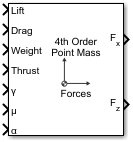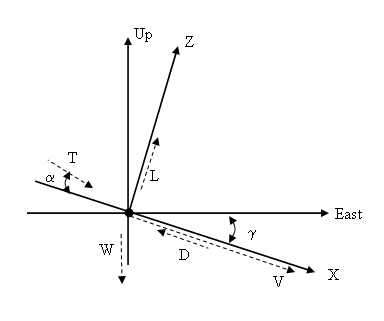# 4th Order Point Mass Forces (Longitudinal)

Calculate forces used by fourth-order point mass

• Library:
• Aerospace Blockset / Equations of Motion / Point Mass

•## Description

The 4th Order Point Mass Forces (Longitudinal) block calculates the applied forces for a single point mass or multiple point masses. For more information on the system for the applied forces, see Algorithms.

## Limitations

The flat Earth reference frame is considered inertial, an approximation that allows the forces due to the Earth motion relative to the "fixed stars" to be neglected.

## Ports

### Input

expand all

Lift, specified as a scalar or array, in units of force.

Data Types: `double`

Drag, specified as a scalar or array, in units of force.

Data Types: `double`

Weight, specified as a scalar or array, in units of force.

Data Types: `double`

Thrust, specified as a scalar or array, in units of force.

Data Types: `double`

Flight path angle, specified as a scalar or array, in radians.

Data Types: `double`

Bank angle, specified as a scalar or array, in radians.

Data Types: `double`

Angle of attack, specified as a scalar or array, in radians.

Data Types: `double`

### Output

expand all

Force in x-axis, returned as a scalar or array, in units of force.

Data Types: `double`

Force in z-axis, returned as a scalar or array, in units of force.

Data Types: `double`

## AlgorithmsThe applied forces [Fx Fz]T are in a system defined as follows: x-axis is in the direction of vehicle velocity relative to air, z-axis is upward, and y-axis completes the right-handed frame. They are functions of lift (L), drag (D), thrust (T), weight (W), flight path angle (γ), angle of attack (α), and bank angle (μ).

`$\begin{array}{l}{F}_{z}=\left(L+T\mathrm{sin}\alpha \right)\mathrm{cos}\mu -W\mathrm{cos}\gamma \\ {F}_{x}=T\mathrm{cos}\alpha -D-W\mathrm{sin}\gamma \end{array}$`

## Extended Capabilities

### C/C++ Code GenerationGenerate C and C++ code using Simulink® Coder™.

Introduced before R2006a

## SupportGet trial now